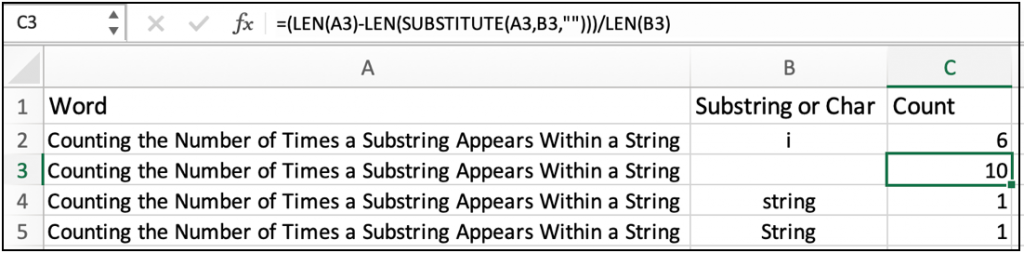# How to Count the Number of Times a Substring Appears Within a String in ExcelIn this article, we will learn How to Count the Number of Times a Substring Appears Within a String in Excel.

Scenario:

Working with excel text values, it's easy and convenient to use excel formulas. For example if we need to find the number of occurrences of a string in a given text in excel "p" in "Apple" are 2. For counting like these we use some basic logic and mathematical formulation to get the result with efficiency. Hope you learn something new out of it.

Count of string in text formula in Excel

Here the logic is we replace all the occurrences of substring in text and the difference of the length of the old text value with new text value will give you the number of occurrences. We can replace the substring with blank space "". Below is the detailed formula

Count of string in text formula

 =(LEN(text)-LEN(SUBSTITUTE(text,substring,"")))/LEN(subtring)

text : text value

substring : value to find

"" : blank space

Note : The above formula is case sensitive, so "p" in "Apple" will be 2 but  "P" in "Apple" will be zero.

Example :

All of these might be confusing to understand. Let's understand how to use the function using an example. Here we have some data to work on text values. The problem is similar as explained above. Given a text and we need to find the number of occurrences. First we calculate the occurrence of "i" in "Counting the Number of Times a Substring Appears Within a String". Use the below formula as mentioned

Use the formula:

 =(LEN(A2)-LEN(SUBSTITUTE(A2,B2,"")))/LEN(B2)

The answer is 6. You can now find any string or char in a full text in excel. IN Cell B2 there is a Space Character (it's not empty).As you can see from the above snapshot that there are 10 single space characters in the given phrase.

You must be noticing the why the both last results give 1 as answer not 2 because "string" and "String" are two different substrings when using in case sensitive formula.

Here are all the observational notes using the formula in Excel
Notes :

1. The formula returns #DIV/0! Error if the substring cell is empty.
2. The above formula is case sensitive, so "p" in "Apple" will be 2 but  "P" in "Apple" will be zero.
3. The formula returns 0 if there is no occurrence of    substring in text.

Hope this article about How to Count the Number of Times a Substring Appears Within a String in Excel is explanatory. Find more articles on calculating values and related Excel formulas here. If you liked our blogs, share it with your friends on Facebook. And also you can follow us on Twitter and Facebook. We would love to hear from you, do let us know how we can improve, complement or innovate our work and make it better for you. Write to us at info@exceltip.com.

Related Articles :

COUNTIFS with Dynamic Criteria Range : Learn to Count cells matching from range based on a dependent cell in Excel here.

COUNTIFS Two Criteria Match : Learn to Count cells matching two different criteria on list in excel here.

COUNTIFS With OR For Multiple Criteria : Learn to Count cells having multiple criteria match using the OR function.

The COUNTIFS Function in Excel : Learn to Count cells dependent on other cell values.

How to Use Countif in VBA in Microsoft Excel : Learn to Count cells using Visual Basic for Applications code.

Use the SUMPRODUCT function to count in Excel : Learn SUMPRODUCT function to Return the count from an array having different criterias in excel here.

How to use wildcards to count matching strings in excel : Learn to Count cells matching phrases, substring or even a character using the formulas with explained wildcards in excel here.

How to use excel Formula to Sort Text : To sort text values using formula in excel we simply use the COUNTIF function. Here is the formula explained.

Popular Articles :